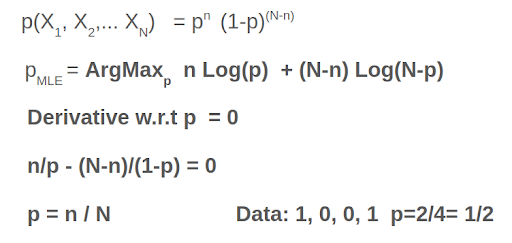# What is the Maximum Likelihood Estimate (MLE)?

Probabilistic Models help us capture the inherant uncertainity in real life situations. Examples of probabilistic models are Logistic Regression, Naive Bayes Classifier and so on..  Typically we fit (find parameters) of such probabilistic models from the training data, and estimate the parameters. The learnt model can then be used on unseen data to make predictions.

One way to find the parameters of a probabilistic model (learn the model) is to use the MLE estimate or the maximum likelihood estimate.

Maximum likelihood estimate is that value for the parameters that maximizes the likelihood of the data.

What are some examples of the parameters of models we want to find? What exactly is the likelihood? How do we find parameters that maximize the likelihood? These are some questions answered by the video.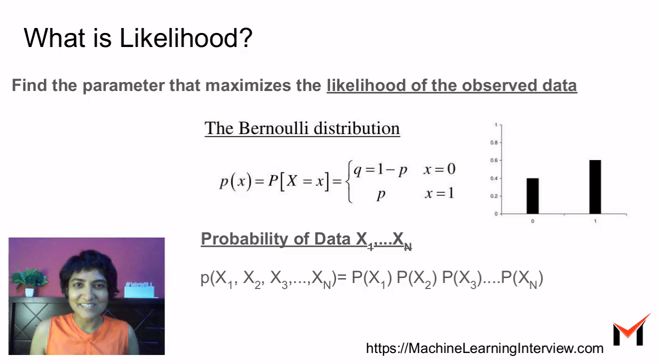##### What are some examples of parameters we might want to estimate?

Consider the Gaussian distribution. The mathematical form of the pdf is shown below. The parameters of the Gaussian distribution are the mean and the variance (or the standard deviation). Given a set of points, the MLE estimate can be used to estimate the parameters of the Gaussian distribution.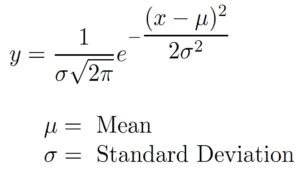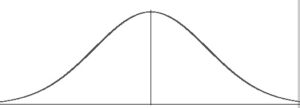Consider the Bernoulli distribution. A good example to relate to the Bernoulli distribution is modeling the probability of heads (p) when we toss a coin. The probability of heads is p, the probability of tails is (1-p). By observing a bunch of coin tosses, one can use the maximum likelihood estimate to find the value of p.

##### What is the likelihood of a probabilistic model?

The likelihood is the joined probability distribution of the observed data given the parameters.

For instance, if we consider the Bernoulli distribution for a coin toss with probability of heads as p. Suppose we toss the coin four times, and get H, T, T, H.

The likelihood of the observed data is the joint probability distribution of the observed data. Hence: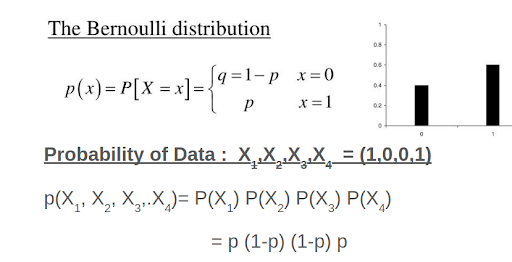##### How do we find the parameter that maximizes likelihood?

The MLE estimator is that value of the parameter which maximizes likelihood of the data. This is an optimization problem.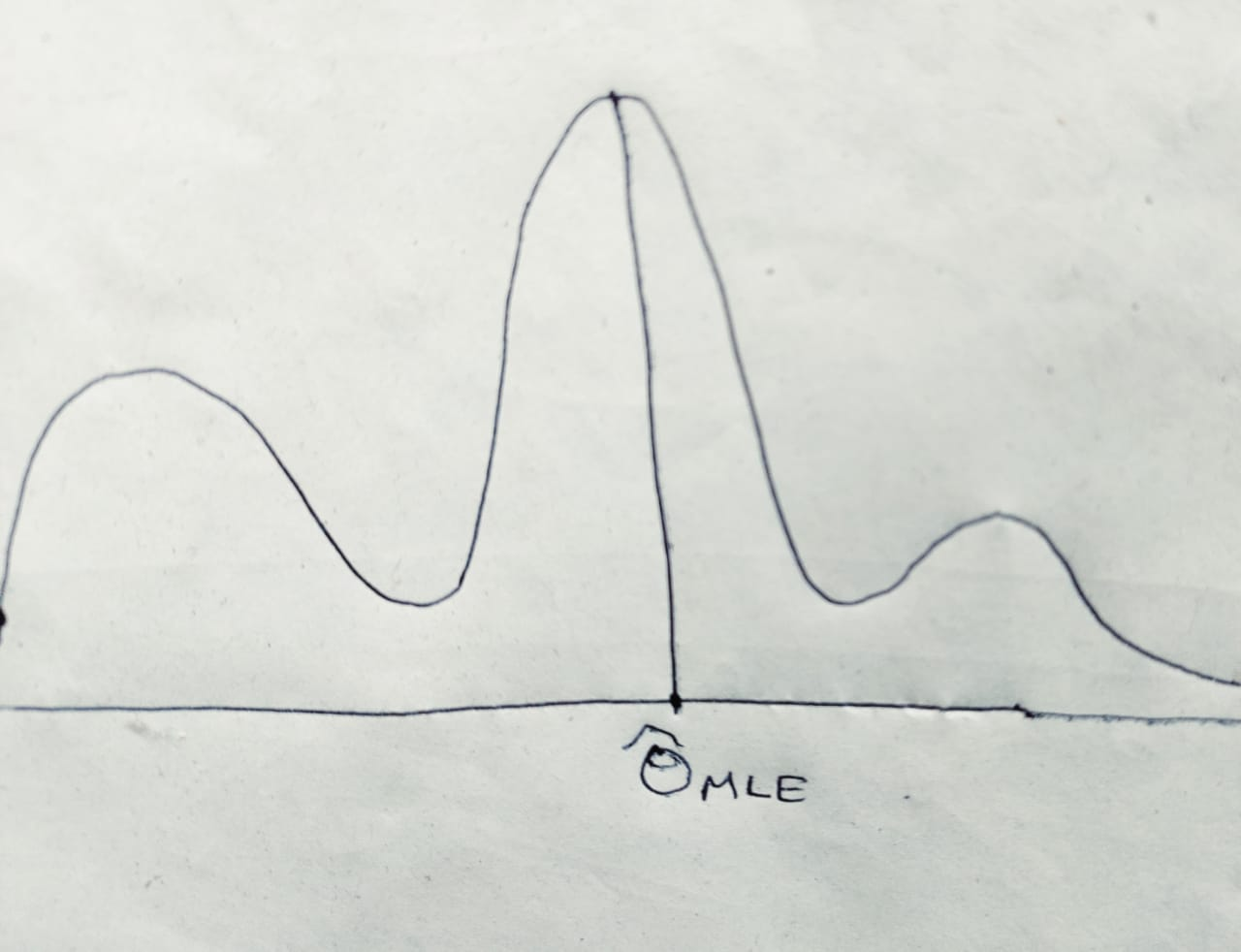For instance for the coin toss example, the MLE estimate would be to find that p such that  p (1-p) (1-p) p is maximized.